# Problem 1: An angle modulated waveform is given as s(t)=A_cos (1000 at +8071 +3204) where 0.=10001...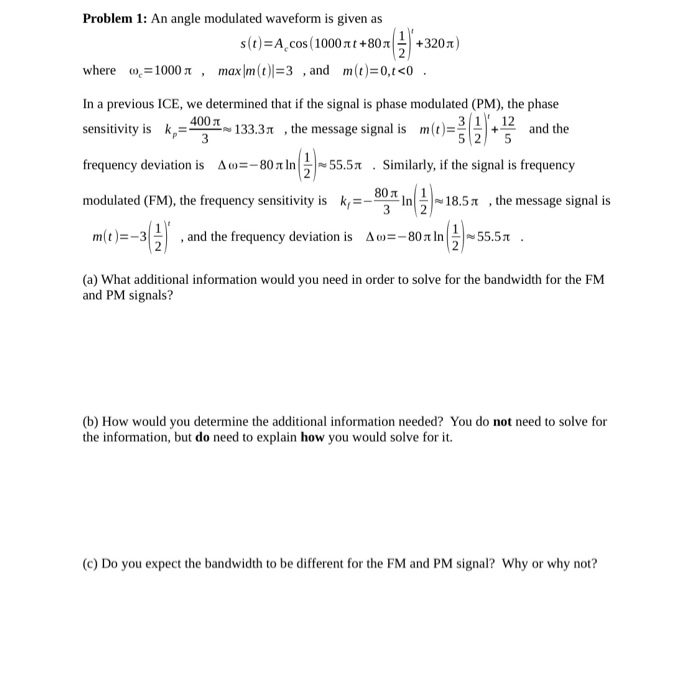Problem 1: An angle modulated waveform is given as s(t)=A_cos (1000 at +8071 +3204) where 0.=10001 , max|m(t)=3 , and m(t)=0,+<0. In a previous ICE, we determined that if the signal is phase modulated (PM), the phase sensitivity is k= 4001 - 133.31 , the message signal is m(t)=1 12 and the frequency deviation is Am=-80 tn 5-55.51 . Similarly, if the signal is frequency modulated (FM), the frequency sensitivity is kr , the message signal is m(t)==3(3), and the frequency deviation is Aw=- 80 In () – 55.51 (a) What additional information would you need in order to solve for the bandwidth for the FM and PM signals? (b) How would you determine the additional information needed? You do not need to solve for the information, but do need to explain how you would solve for it. (c) Do you expect the bandwidth to be different for the FM and PM signal? Why or why not?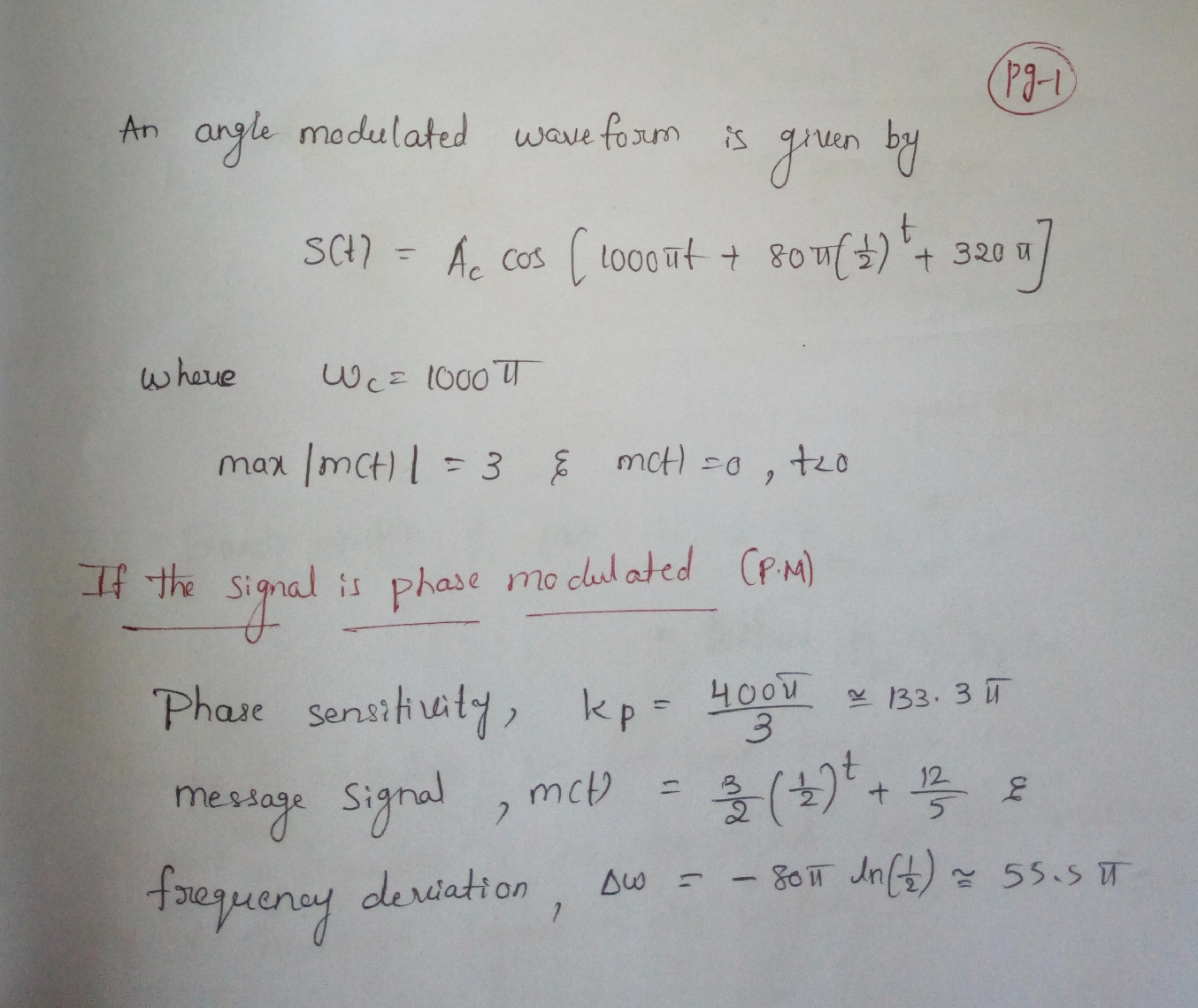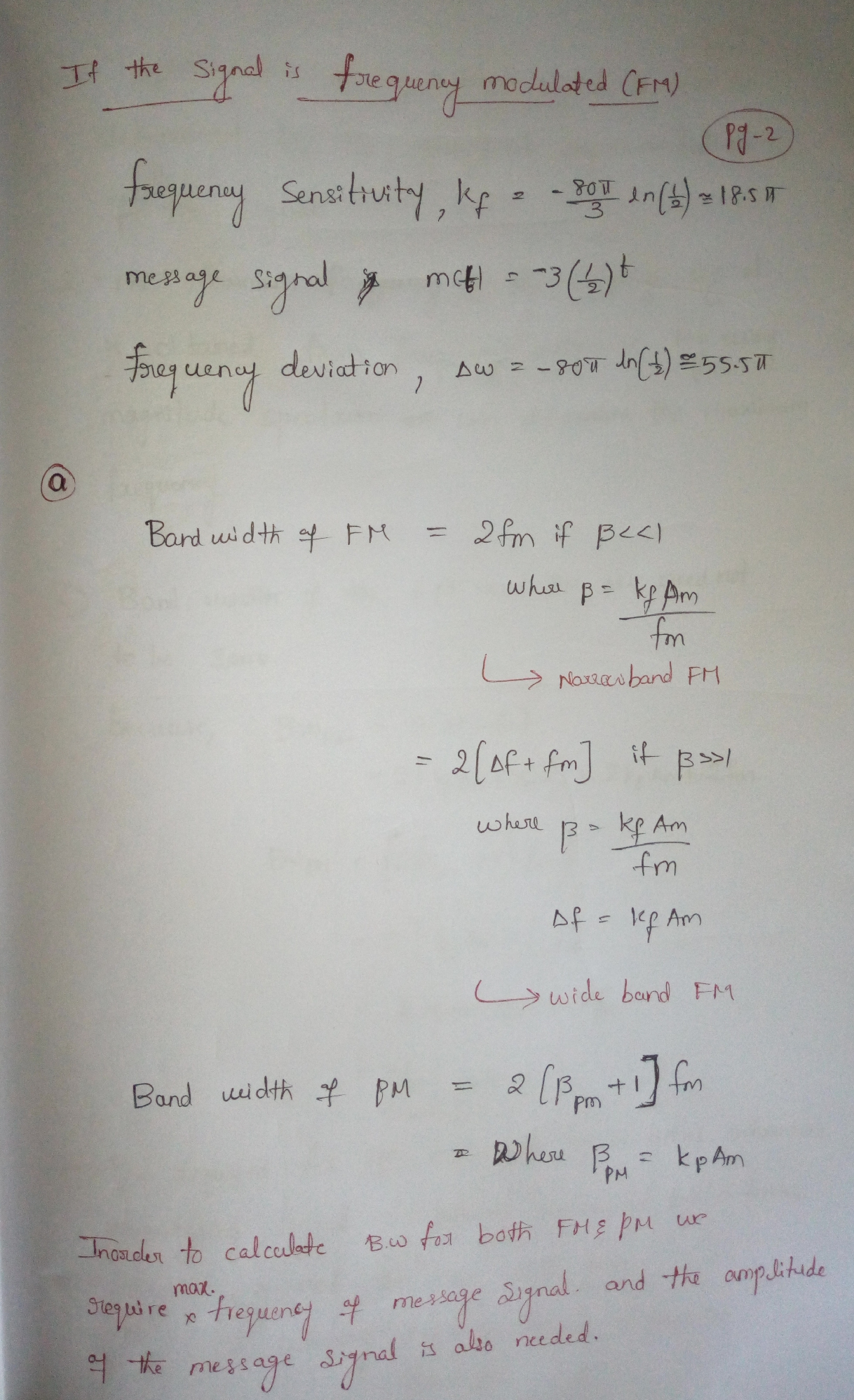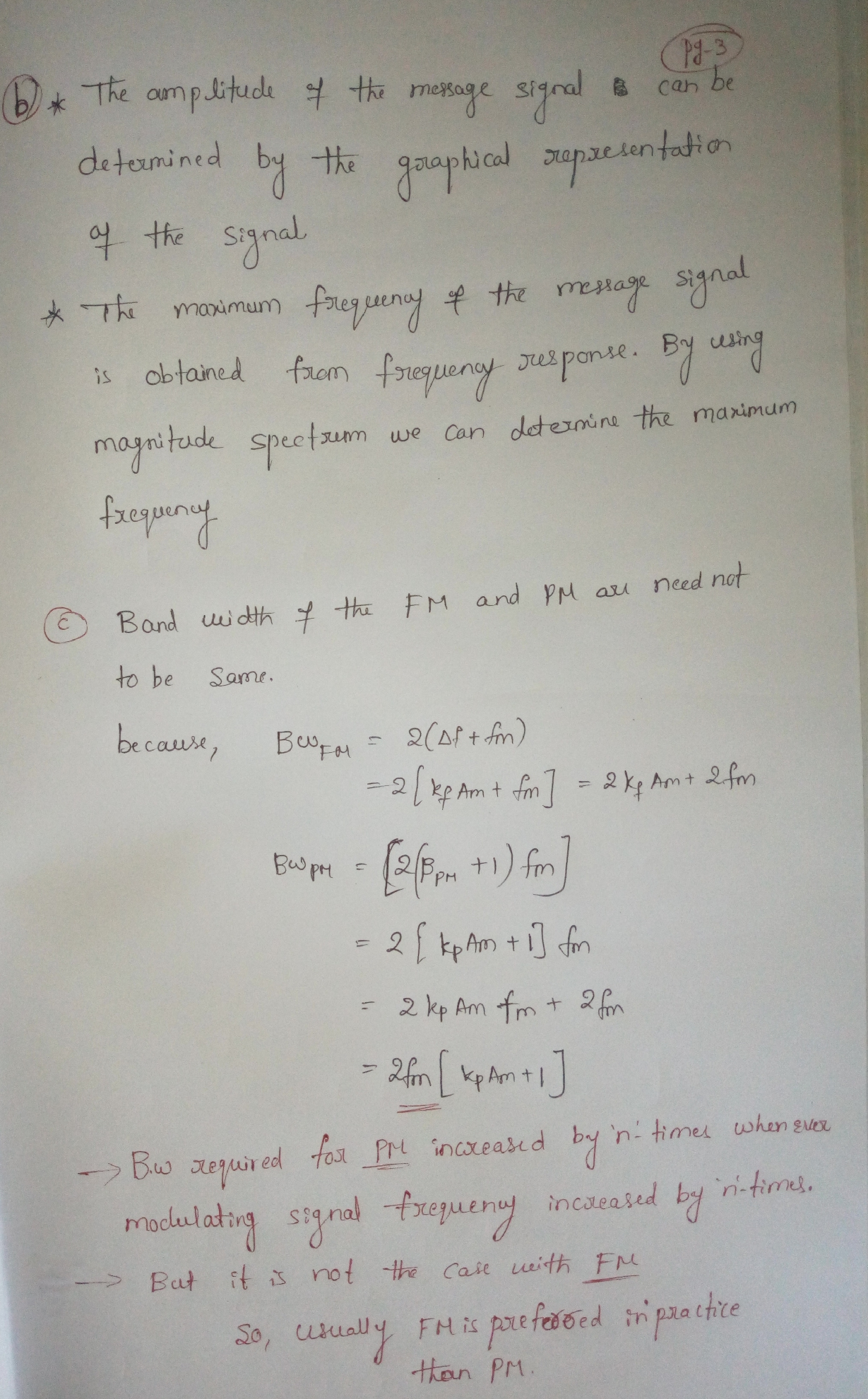##### Add Answer of: Problem 1: An angle modulated waveform is given as s(t)=A_cos (1000 at +8071 +3204) where 0.=10001...
Similar Homework Help Questions
• ### Find he 20 200 114 pts) Problem 6) A phase-modulated signal is denoted by the following equation: (a) Find the power of the modulated signal. (b) Find the carrier frequency fe (c) Find the f...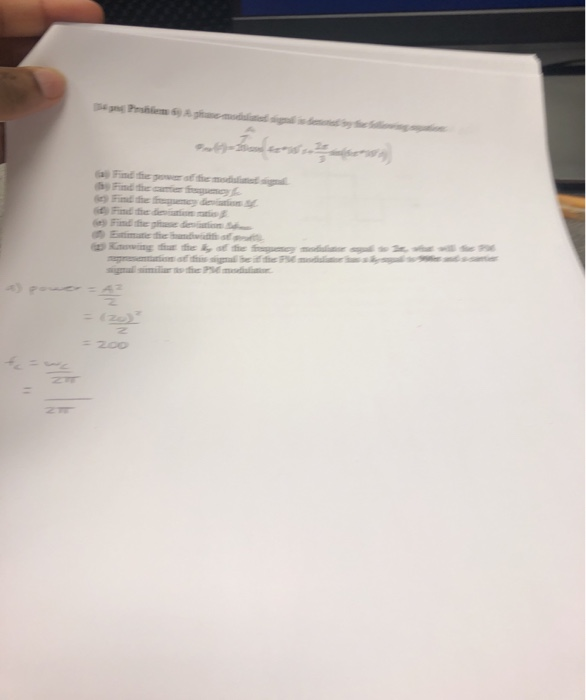Find he 20 200 114 pts) Problem 6) A phase-modulated signal is denoted by the following equation: (a) Find the power of the modulated signal. (b) Find the carrier frequency fe (c) Find the frequency deviation Δ. (d) Find the deviation ratio B (e) Find the phase deviation A (t Estimate the bandwidth of pptt). (g) Knowing that the k, of the frequency modulator equal to 2n, what will the FM representation of this signal be if the FM modulator...

• ### 5. Consider the following message signal: m(t) -2 .3 10 Assume that the carrier frequency is f ar...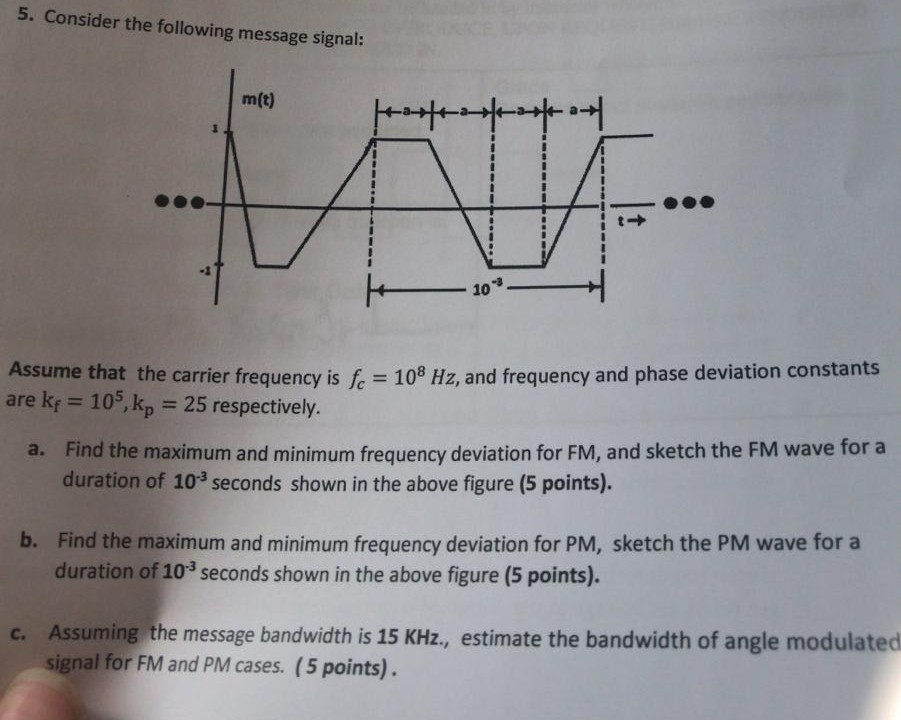5. Consider the following message signal: m(t) -2 .3 10 Assume that the carrier frequency is f are kf = 105, kp-25 respectively. 108 Hz, and frequency and phase deviation constants Find the maximum and minimum frequency deviation for FM, and sketch the FM wave for a duration of 103 seconds shown in the above figure (5 points). a. b. Find the maximum and minimum frequency deviation for PM, sketch the PM wave for a duration of 103 seconds shown...

• ### 5-20pts) Let s(t) be an angle-modulated signal that a receiver obtains. s(t) 2cos(10'rt +2sin(200...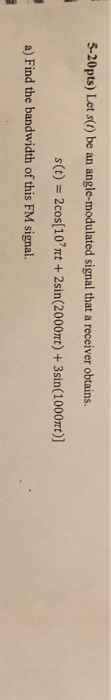5-20pts) Let s(t) be an angle-modulated signal that a receiver obtains. s(t) 2cos(10'rt +2sin(2000mt)+3sin(1000mt)] a) Find the bandwidth of this FM signal. b) If s() is sent to an (ideal) envelope detector, find the detector output signal. c) If s(t) is first differentiated before the envelope detector, find the detector output signal. d) Explain which detector output can be processed to yield the message signal m() and find the message signal m() if ky- 200π. 5-20pts) Let s(t) be an...

• ### An angle-modulated signal is given by: x(t) = Acos(2fsnt + Bcos(2fm Tt)) Find the instantaneous frequency,...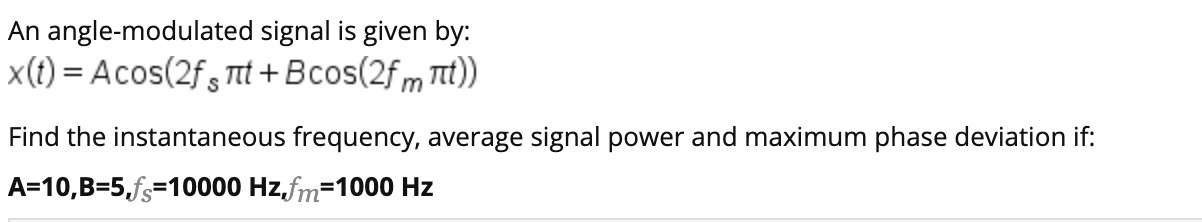An angle-modulated signal is given by: x(t) = Acos(2fsnt + Bcos(2fm Tt)) Find the instantaneous frequency, average signal power and maximum phase deviation if: A=10,B=5,fs=10000 Hz,fm=1000 Hz

• ### 1.) Angle Modulation can be subdivided into phase modulation (PM) and frequency modulation (FM). Highlight the...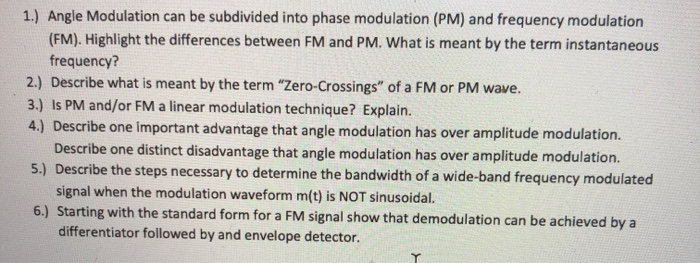1.) Angle Modulation can be subdivided into phase modulation (PM) and frequency modulation (FM). Highlight the differences between FM and PM. What is meant by the term instantaneous frequency? 2.) Describe what is meant by the term "Zero-Crossings" of a FM or PM wave. 3.) Is PM and/or FM a linear modulation technique? Explain. 4.) Describe one important advantage that angle modulation has over amplitude modulation. Describe one distinct disadvantage that angle modulation has over amplitude modulation. 5.) Describe the...

• ### This problem is on the basics of FM using single tone message. As we that certain...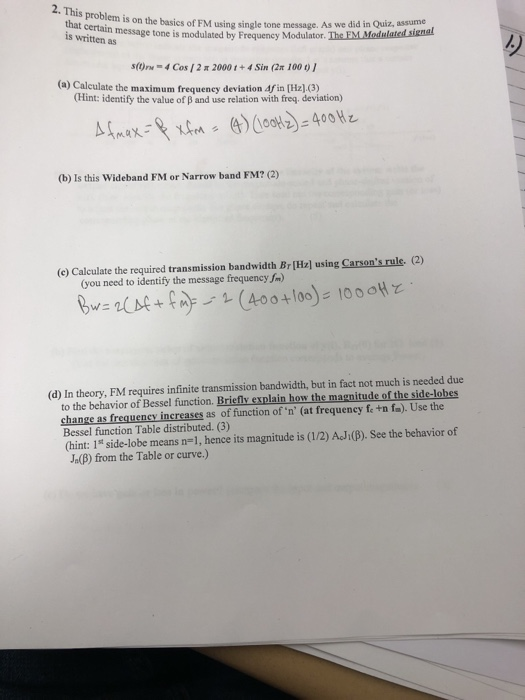This problem is on the basics of FM using single tone message. As we that certain message tone is modulated by Frequency Modulator is written as s(t)r'. 4 Cos [ 2 π 2000 t + 4 Sin (2x 10001 (a) Caleulate the maximum frequency deviation dfin (Hz](3) Hint: identify the value of B and use relation with freq. deviation) (b) Is this Wideband FM or Narrow band FM? (2) (c) Calculate the required transmission bandwidth Brl㎐l using Carson's rule (2)...

• ### Consider a FM system that is used to communicate the following message signal: S. m(t) 20...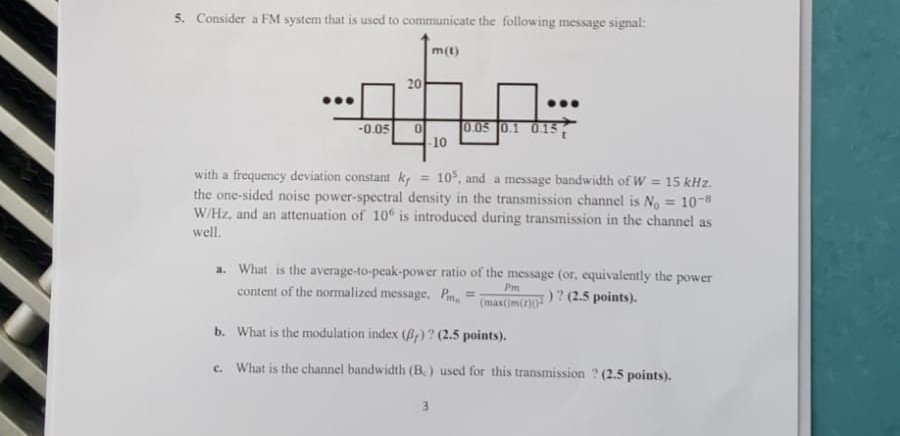Consider a FM system that is used to communicate the following message signal: S. m(t) 20 0.05 0.1 015 10 -0.05 with a frequency deviation constant ky 10, and a message bandwidth of W =15 kHz the one-sided noise power-spectral density in the transmission channel is No= 10-8 W/Hz, and an attenuation of 10 is introduced during transmission in the channel as well. a. What is the average-to-peak-power ratio of the message (or, equivalently the power content of the normalized...

• ### Consider a FM system that is used to communicate the following message signal: S. m(t) 20...Consider a FM system that is used to communicate the following message signal: S. m(t) 20 0.05 0.1 015 10 -0.05 with a frequency deviation constant ky 10, and a message bandwidth of W =15 kHz the one-sided noise power-spectral density in the transmission channel is No= 10-8 W/Hz, and an attenuation of 10 is introduced during transmission in the channel as well. a. What is the average-to-peak-power ratio of the message (or, equivalently the power content of the normalized...

• ### 1. Given a baseband signal m(t) sin(1000mt) cos(3000nt) + cos(3700nt a. Sketch the spectrum of m(...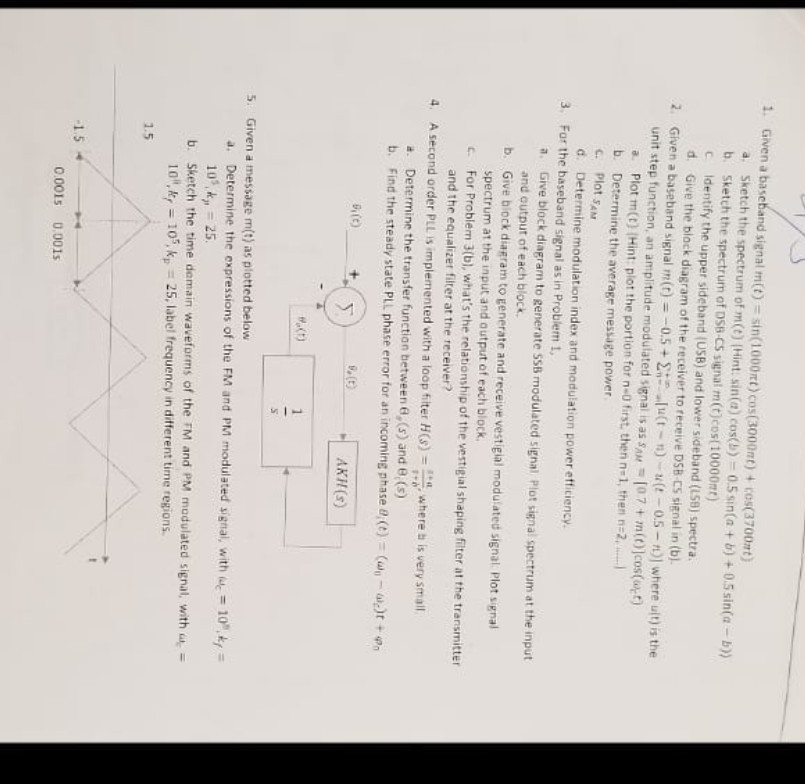1. Given a baseband signal m(t) sin(1000mt) cos(3000nt) + cos(3700nt a. Sketch the spectrum of m(t) (Hint. sin(a) cos(b) 0.5 sin(a +b) +0.5sin a-b)) b. Sketch the spectrum of DSB-CS signal m(t)cos(10000mt) C ldentify the upper sideband {USB) and lower sideband (LSB) spectra d. Give the black diagram of the receiver to receive DSB-CS signal in (b). 2. baseband signal m(r)--0.5 + Σ..小(t-n)-u(t-0.5-n)] where ult) is the Given unit step function, an amplitude modulated signal is as SAM 107+ m(0cos...

• ### 5) given the following PM modulated signal equation, determine the original message equation, m(t) . s(t)=...

5) given the following PM modulated signal equation, determine the original message equation, m(t) . s(t)= 3.5cos(2π2E9t + 0.5cos(2π6000t)), Kp=0.1 (hz/v) a) m(t)=0.16cos(2π6000t) b) m(t) = 5cos(2π6E3t) c) m(t) = 5cos(2π2E9t) d) m(t) = 0.16cos(2π2.4E9t) 6) given the following message and carrier waves. Determine the AM signal equation and (DSB-LC) bandwidth: m(t) =2sin(2π10KHz*t). c(t) =3sin(2π2.4E9Hz*t) a) 5[1+0.6sin(200πt)]sin(2π10E9t), BW=10kHz b) 3[1+0.67 sin(2π10E3t)]sin(2π2.4E9t), BW=20kHz c) 3[1+0.6sin(2.4GHz*πt)]sin(2π10E3Hz*t), BW=20kHz d) 3[1+1.67 sin(2π50t)]sin(2π10E9tt), BW=10kHz 7) amplitude used in a communication path will increase both signal...

Free Homework App